## Predicting access of Amazon employees: Deep learning- Data analysis

There is a considerable amount of data regarding an employee’s role within an organization and the resources to which they have access. Here I have predicted whether to give access or not to a particular employee.

Introduction

Amazon is an American multinational company whose business includes cloud computing, e-commerce, artificial intelligence, consumer electronics, digital distribution, grocery stores. When an employee at any company starts work, they first need to obtain the computer access necessary to fulfill their role. This access may allow an employee to read/manipulate resources through various applications or web portals. It is assumed that employees fulfilling the functions of a given role will access the same or similar resources. It is often the case that employees figure out the access they need as they encounter roadblocks during their daily work (e.g. not able to log into a reporting portal). A knowledgeable supervisor then takes time to manually grant the needed access in order to overcome access obstacles. As employees move throughout a company, this access discovery/ recovery cycle wastes a nontrivial amount of time and money.

There is a considerable amount of data regarding an employee’s role within an organization and the resources to which they have access. Given the data related to current employees and their provisioned access, models can be built that automatically determine access privileges as employees enter and leave roles within a company. These auto-access models seek to minimize the human involvement required to grant or revoke employee access.

This is a Kaggle dataset competition and the objective of this competition is to build a model, learned using historical data, that will determine an employee's access needs, such that manual access transactions (grants and revokes) are minimized as the employee's attributes change over time. The model will take an employee's role information and a resource code and will return whether access should be granted. Different models including Logistic regression model, stochastic gradient descent model, Support vector machine model and neural network models are used to predict whether employee will be granted access or not. This is a binary classification prediction.

Data collection

The data consists of real historical data collected from 2010 2011.  Employees are manually allowed or denied access to resources over time. You must create an algorithm capable of learning from this historical data to predict approval/denial for an unseen set of employees. The entire dataset consists of around 100K employee records.

File Descriptions:

csv - The training set. Each row has the ACTION (ground truth), RESOURCE, and information about the employee's role at the time of approval. This file consists of 32770 employee records.

csv - The test set for which predictions should be made.  Each row asks whether an employee having the listed characteristics should have access to the listed resource. This file consists of 58922 records.

Column Descriptions:

 Column Name Description ACTION ACTION is 1 if the resource was approved, 0 if the resource was not RESOURCE An ID for each resource MGR_ID The EMPLOYEE ID of the manager of the current EMPLOYEE ID record; an employee may have only one manager at a time ROLE_ROLLUP_1 Company role grouping category id 1 (e.g. US Engineering) ROLE_ROLLUP_2 Company role grouping category id 2 (e.g. US Retail) ROLE_DEPTNAME Company role department description (e.g. Retail) ROLE_TITLE Company role business title description (e.g. Senior Engineering Retail Manager) ROLE_FAMILY_DESC Company role family extended description (e.g. Retail Manager, Software Engineering) ROLE_FAMILY Company role family description (e.g. Retail Manager) ROLE_CODE Company role code; this code is unique to each role (e.g. Manager)

## 3.     Data Exploration

The step in the machine learning process after collecting the data involves examining data in detail. To solve machine learning algorithms, it is important to understand the dataset. Below is the detailed description of the dataset.

Training dataset: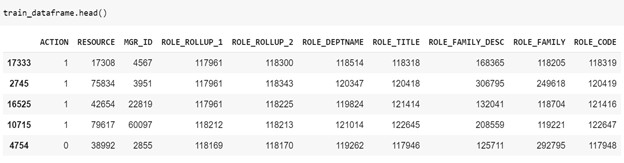Test dataset: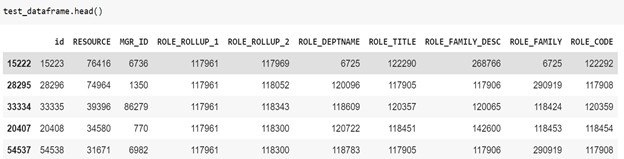Checking the shape of data frames:

As shown in the shape of data frame, dataset has 10 columns and total 91690 records. The target column contains binary values which are 0 and 1. Below is the distribution of ACTION column.

As shown in the figure above, there are 1897 records which belongs to ‘0’ and 30872 records which belongs to ‘1’ in ACTION category.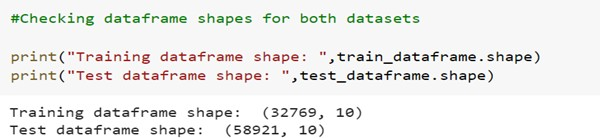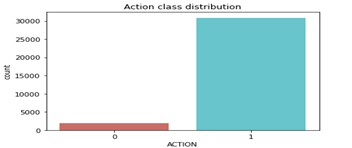Data Preprocessing

Handling Missing values:

After exploring data, the next step is to check if there are any missing values in the dataset. Missing data is everyday problem of data scientist; hence it is important to find if there are any missing values.

Below is the description of the null values. As shown in the figure below, there are no null values in the dataset. Therefore, there is no need to change or eliminate anything (use missing handling techniques) at this step.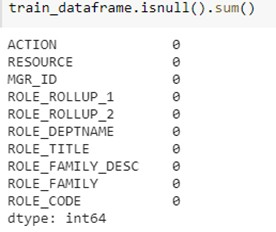Handling Outliers:

After checking null values, it is important to check if there are any outliers in the dataset. Outliers are data points that do not belong to certain population. An outlier is an observation that diverges from otherwise well-structured data.

As the dataset contains only categorical and binary data, there is no need to check outliers as categiorical data means It's just the composition of the sample which   has been selected.

Feature Selection:

To transform the data, it is important to understand categories and distribution of each variable. As the dataset contains categorical and binary values let’s check how many categories are there in each column. Below are the unique values which data contains: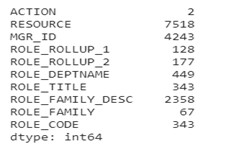Figure: Unique values of all variables

From above values, we can see that except from ACTION variable all other variables have more than 2 categories. Let’s see how the distribution of each variable in dataset from below figure is. I used seaborn distplot () to visualize the distribution of features in the dataset.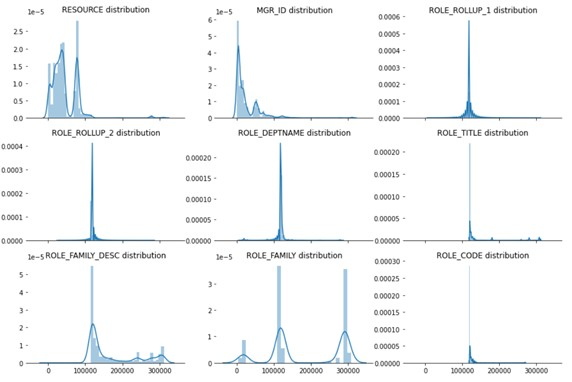Figure: Distribution of all variables

Using above analysis, I can see that ROLE_TITLE and ROLE_CODE have exact same distribution. Hence, I can drop one of the columns from those two, but if we check values of categories, they are not the same. Therefore, it might not be the same hence, I will not be dropping any column in this step.

Let’s check correlation between target variable with feature variables. I am using correlation matrix with heat map for better visualization. The heat map of correlation as shown in the figure below: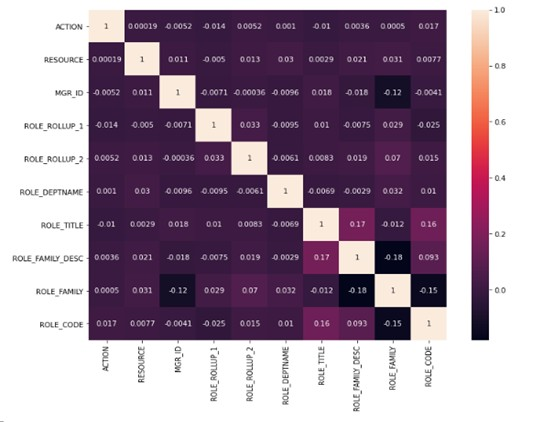Figure: Heat map of correlation values

As shown in the figure above of heat map of correlation, all the variables have weak correlation or negative correlation. Therefore, it will be fun to analyze data which do not have strong dependency/weak correlation on the target variable. As shown in the unique value figure, ROLE_TITLE and ROLE_CODE have exact same distribution. Let’s check correlation between them.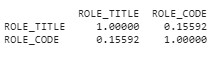When I checked the correlation between ROLE_CODE and ROLE_TITLE, they have weak correlation hence I will not be dropping any variable in the feature selection process.

Data Transformation

1. Data Transform:

All the variables in the dataset are categorical variables. The categorical value represents the numerical value of the entry in the dataset. One hot encoding allows categorical data to be more expressive hence, I will use a one-hot encoder with fit_transform () to encode, transform, and fit the data. I am applying this on the training and test set of data, I have used fit_transform () on test data set as well so I can have sparse matrix while predicting the test data.

Fit_transform () joins the fit () and transform () method for transformation of dataset. The input to this transformer should be a matrix of integers, denoting the values taken on by categorical (discrete) features. And The output will be a sparse matrix where each column corresponds to one possible value of one feature.

The results of data frame after applying one hot encoding is as below. As shown in the figure below, I have sparse matrix for training and testing data frames and training target variable consists with 0 and 1 values which indicates status of access given to the employees.

Data Split:

The dataset consists of two main csv files train and test. For checking the accuracy of the models, we need validation data frame on which we can check the accuracy of that model. Hence, it is important to split the training dataset. Once the model has good accuracy, then we can use that model to predict test dataset values on whether to give access to the employees or not. Once I split the data, I will have following data frames:

Training Dataset: The sample of data used to fit the model.

Validation Dataset: The sample of data used to provide an unbiased evaluation of a model fit on the training dataset while tuning model hyperparameters. The evaluation becomes more biased as skill on the validation dataset is incorporated into the model configuration.

Test Dataset: The sample of data used to provide an unbiased evaluation of a final model fit on the training dataset.

The shape of all data frames after splitting the data is as shown below. Now, we can use these data frames in our model.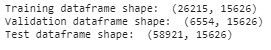Building the Models

After data pre-processing, next step is to build different classification models to predict the test data. The purpose of building the models is to predict the values of ACTION variable to check the employee should be given access or not for the test dataset.

Logistic Regression Model:

Building the model:

Logistic regression model is used to predict the target variable which is categorical variable. Logistic regression is a predictive analysis. Logistic regression is used to describe data and to explain the relationship between one dependent binary variable and one or more nominal, ordinal, interval or ratio-level independent variables.

After building the model, it is important to check the score and summary of the model to check the validity of the model. Below is the Model summary of Logistic regression model.

Model fitting:

We can use fit () method to fit this logistic regression model to data. Later, we can use this fitted model to predict the data. From this we can use the output of this method to find the accuracy of the model. The validation accuracy of the logistic regression model is 94.74% and training accuracy is 96.03%.

Model evaluation:

Next step is to check the accuracy of the logistic regression model on validation dataset.                           The formula to calculate the misclassification rate is as below,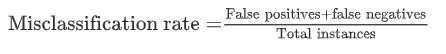Only 5.26% data is misclassified therefore the accuracy of the model is 94.74%.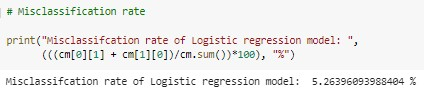Below is the heat map of confusion matrix. From this we can say that, out of all the validation data set 6155 are predicted correctly as target 1 and 54 are predicted correctly as target 0.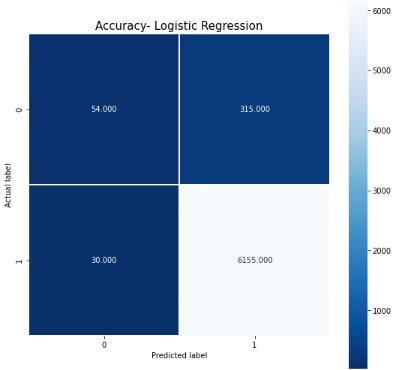Below is the classification report of Logistic regression model.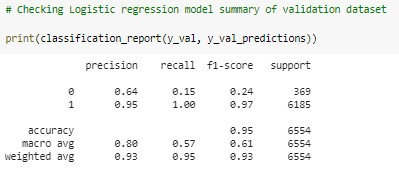Model prediction:

After training the model, evaluating it on validation dataset next step is to predict the test dataset ACTION variable. Below is the result of ACTION variable prediction where I have predicted whether the employee has the access or not.

From the test dataset below is the result of predicted ACTION variable of test data set. Out of which 58037 employees have been given the access and 884 employees will not be given access.

Below is the ROC curve which is used for binary classification. The dotted line represents the ROC curve of a purely random classifier; a good classifier stays as far away from that line as possible (toward the top-left corner). Hence, the logistic regression model gave us good result comparatively.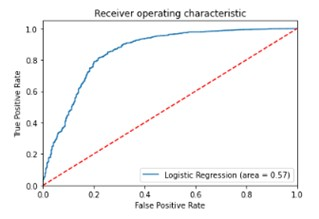Dense Neural Network (DNN) Model:

Building the model:

Machine learning has a popular subset which is deep learning. Deep learning is used to build neural network models. A neural network models have 3 main layers, which are input layer, hidden layer and output layer. The hidden layers use weights which are adjusted during training and then used to predict models. The neural network learns on its own, we don’t have to specify the pattern to look for. I will use this binary classification model to predict whether to give the employee access or not.

After building the model, it is important to check the score and summary of the model to check the validity of the model. Below are the Model summary and model epochs history- accuracy loss summary of DNN Model.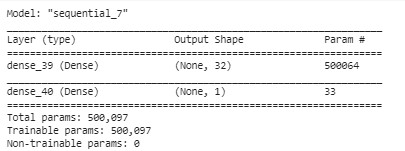DNN Model Summary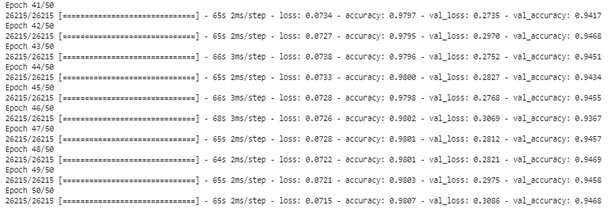DNN Model epochs history- accuracy loss Summary

Model fitting:

We can use fit () method to fit this neural network model to data. Later, we can use this fitted model to predict the data. From this we can use the output of this method to find the accuracy of the model. The accuracy of the neural network model is 94.68% for validation dataset and 98.01% for training dataset.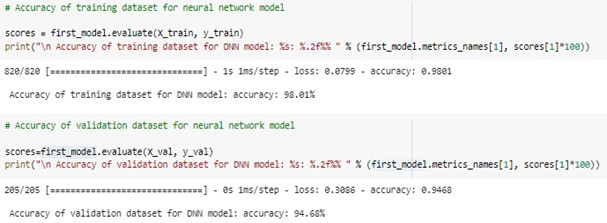Model evaluation:

Next step is to check the accuracy of the Dense neural network model on validation dataset. Below is the DNN Model accuracy map of training and validation accuracies. From this we can say that, generalization gap between training data and validation data is comparatively more and hence we can try improving generalization error between training and validation loss.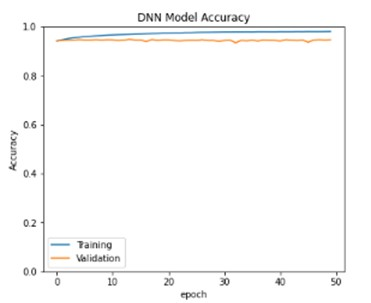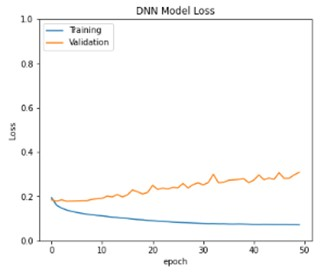Model prediction:

After training the model, evaluating it on validation dataset next step is to predict the test dataset ACTION variable. Below is the result of ACTION variable prediction where I have predicted whether the employee has the access or not.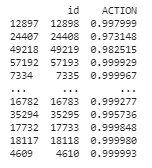Below is the graph of weights of dense neural network model: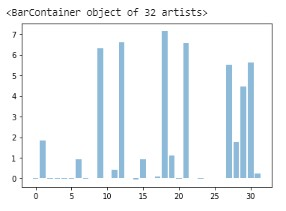Model Comparison and conclusion

After completing the building and fitting all the models, the next step is to find the best model which will be best to predict the output correctly. I have also created a few different models and their results to compare the performance. (These additional models are not described above)

 Models Training Accuracy Validation Accuracy Logistic regression model 96.03 94.74 Stochastic Gradient Descent (SGD) 94.93 94.31 Support Vector Machine (SVM) 95.76 94.54 Dense Neural Network -1 98.01 94.68 Dense Neural Network L1 94.23 94.13

From above models, we can see that we have accuracies in between 94.13 to 98.01. But the generalization error gap is extremely less for Dense neural network L1 model, whereas its greater for Dense neural network model.

This proves that with using regularization techniques we can improve the generalization gap as well as we can get comparatively good accuracy as well. Hence, we are getting better predictions and accuracy with DNN L1 model.

Best model is: DNN L1 with validation accuracy: 94.13%

Therefore, model is the best model for Amazon employee access challenge. Once predicted the output of the best model is saved in the Submission file.

Below is the graphical representation of Model Comparison: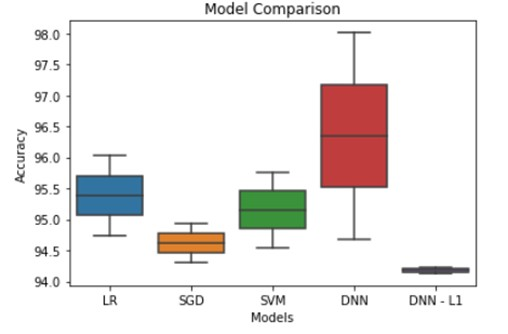After finding the best model, saved all the predictions in submissions.csv file and we got 57211 records as output 1 and 1718 records as value 0.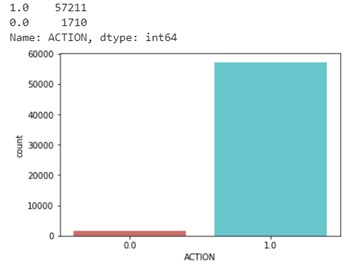References

https://towardsdatascience.com/how-to-handle-missing-data-8646b18db0d4